# 数据的表示：原码、反码、补码、移码以及浮点数的运算_村雨遥

## 什么是 R 进制

`R` 进制数的每一位数值用 R k R^k Rk 的形式表示，即幂底数为 `R`，指数为 `k``k` 与该位和小数点间的间距有关。当该位位于小数点左边时，`k` 则是该位和小数点之间数码的个数；而当该位维语小数点右边时，则 `k` 是负值，其绝对值为该位和小数点之间数码的个数加 `1`

## 进制之间的转换

1. 十进制转 `R` 进制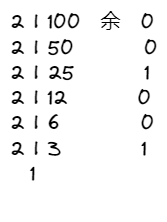1. 二进制转八/十六进制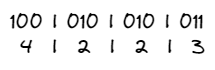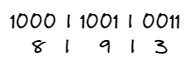## 码制

1-1+0-0

1. 符号位

1. 原码

0001 + 0010 = 0011 ， 1 + 2 = 3 0001 + 0010=0011，1 + 2 = 3 0001+0010=00111+2=3

0000 + 1000 = 1000 ， 0 + ( − 0 ) = − 0 0000 + 1000= 1000，0 + (-0)=-0 0000+1000=10000+(0)=0

0001 + 1001 = 1010 ， 1 + ( − 1 ) = − 2 0001+1001=1010，1+(-1)=-2 0001+1001=10101+(1)=2

1. 反码

• 如果一个数是正数，那么其反码和原码一样，如 1 1 1 的原码和反码均为 0001 0001 0001
• 如果一个数是负数，那么其反码就是其原码除符号位之外，按位取反。如 − 1 -1 1 的原码为 1001 1001 1001，其反码为 1110 1110 1110

0001 + 1001 = 1111 ， 1 + ( − 1 ) = − 0 0001+1001=1111，1+(-1)=-0 0001+1001=11111+(1)=0

1110 + 1100 = 1010 ， ( − 1 ) + ( − 3 ) = − 5 1110+1100=1010，(-1)+(-3)=-5 1110+1100=1010(1)+(3)=5

1. 补码

• 如果一个数是正数，那么该数的补码等于其原码，如 1 1 1 的原码和补码均为 0001 0001 0001
• 如果一个数是负数，那么该数的补码等于反码 + 1 +1 +1，如 − 1 -1 1 的反码为 `1110`，那么其补码即为 `1111`

1111 + 1111 = 1110 ， ( − 1 ) + ( − 1 ) = − 2 1111+1111=1110，(-1)+(-1)=-2 1111+1111=1110(1)+(1)=2

1. 不同码制之间的转换总结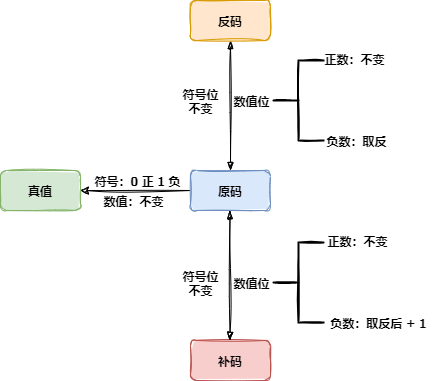1. 移码

1-1+0-0

## 数值表示范围

− ( 2 n − 1 − 1 ) -(2^{n-1}-1) (2n11) ~ + ( 2 n − 1 − 1 ) , − 127   + 127 +(2^{n-1}-1),-127~+127 +(2n11),127 +127

− ( 1 − 2 − ( n − 1 ) ) -(1-2^{-(n-1)}) (12(n1)) ~ + ( 1 − 2 − ( n − 1 ) ) +(1-2^{-(n-1)}) +(12(n1))

## 浮点数的运算

### 浮点数的表示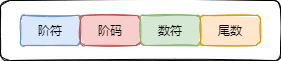N = M ∗ R e N = M * R^e N=MRe

### 浮点数运算

m = 1.0 ∗ 1 0 3 m = 1.0*10^3 m=1.0103

n = 0.25 ∗ 1 0 3 n=0.25*10^3 n=0.25103

## 总结

<< 上一篇 下一篇 >>

### 评论（0） 赞助本站◎欢迎参与讨论，请在这里发表您的看法、交流您的观点。